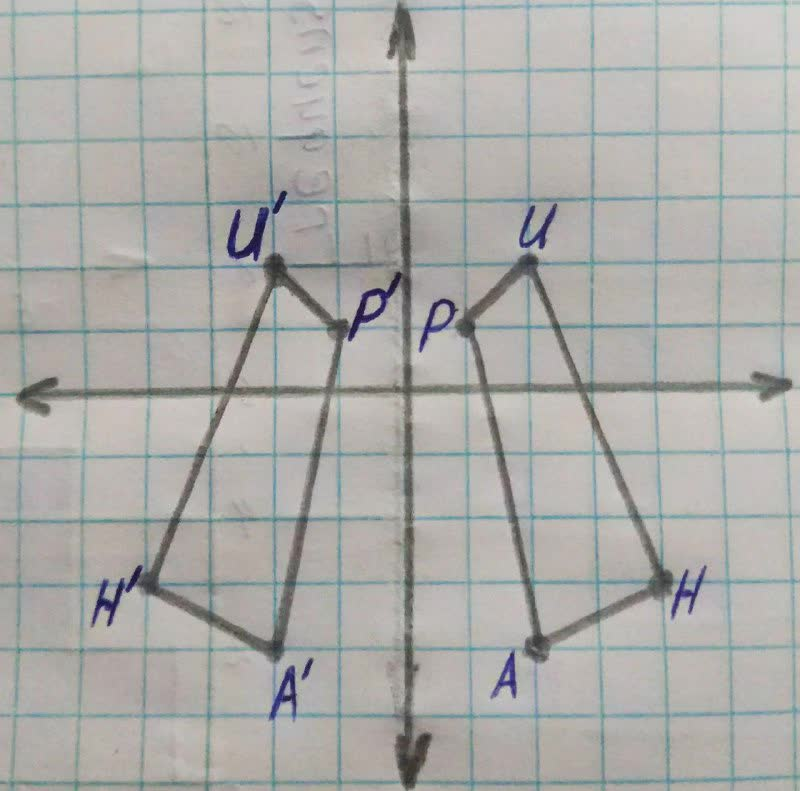# Write a rule to describe each transformation 19610700851.jpgDouglas Kraatz 2021-11-23 Answered
Write a rule to describe each transformation• Questions are typically answered in as fast as 30 minutes

### Plainmath recommends

• Get a detailed answer even on the hardest topics.
• Ask an expert for a step-by-step guidance to learn to do it yourself.Heack1991

Step 1
From the figure, we see that AHUP reflected over the y-axis to form A'H'U'P'.
$$\begin{array}{|c|c|}\hline Old\ coordinates\ & New\ coordinates\ \\ \hline A=(2,-4) & A'=(-2,-4)\\ \hline H=(4,-3 & H'=(-4,-3) \\ \hline U=(2,2) & U'=(-2,2) \\ \hline P=(1,1) & P'=(-1,1)\\ \hline \end{array}$$
From the table we see the x coordinates changes sign.
So we can write a formula as $$\displaystyle{\left({x},{y}\right)}\rightarrow{\left(−{x},{y}\right)}$$

Step 2 Answer: Reflection across the y-axis.
Rule is $$\displaystyle={\left({x},{y}\right)}\rightarrow{\left(−{x},{y}\right)}$$